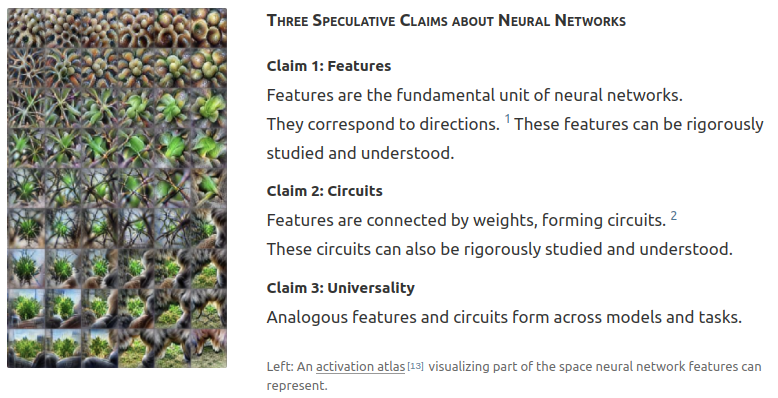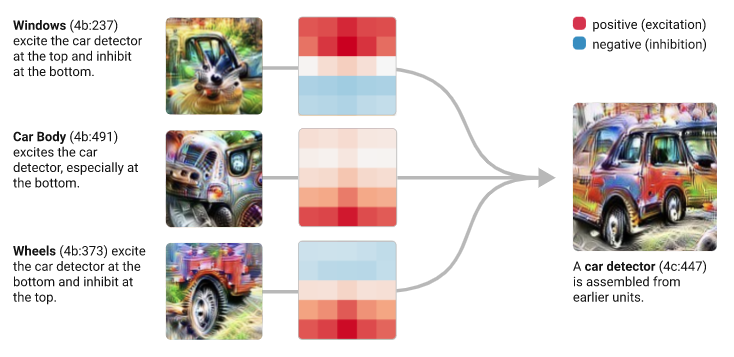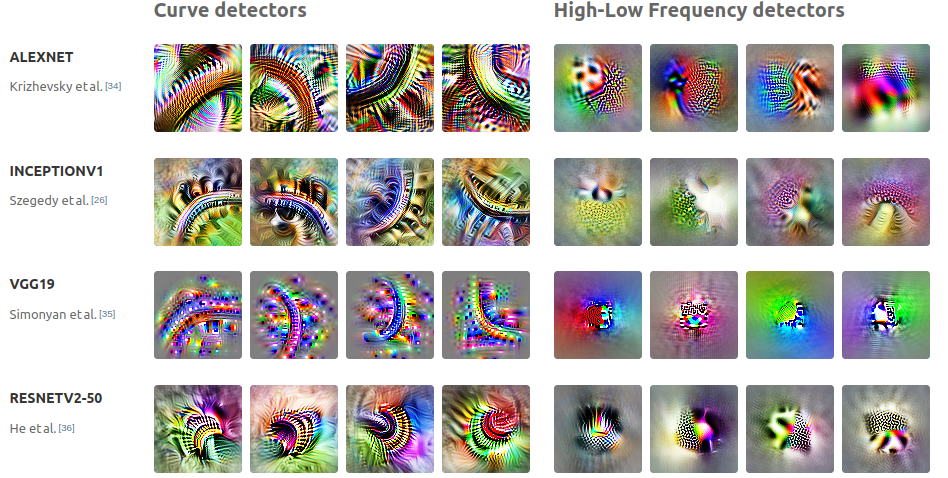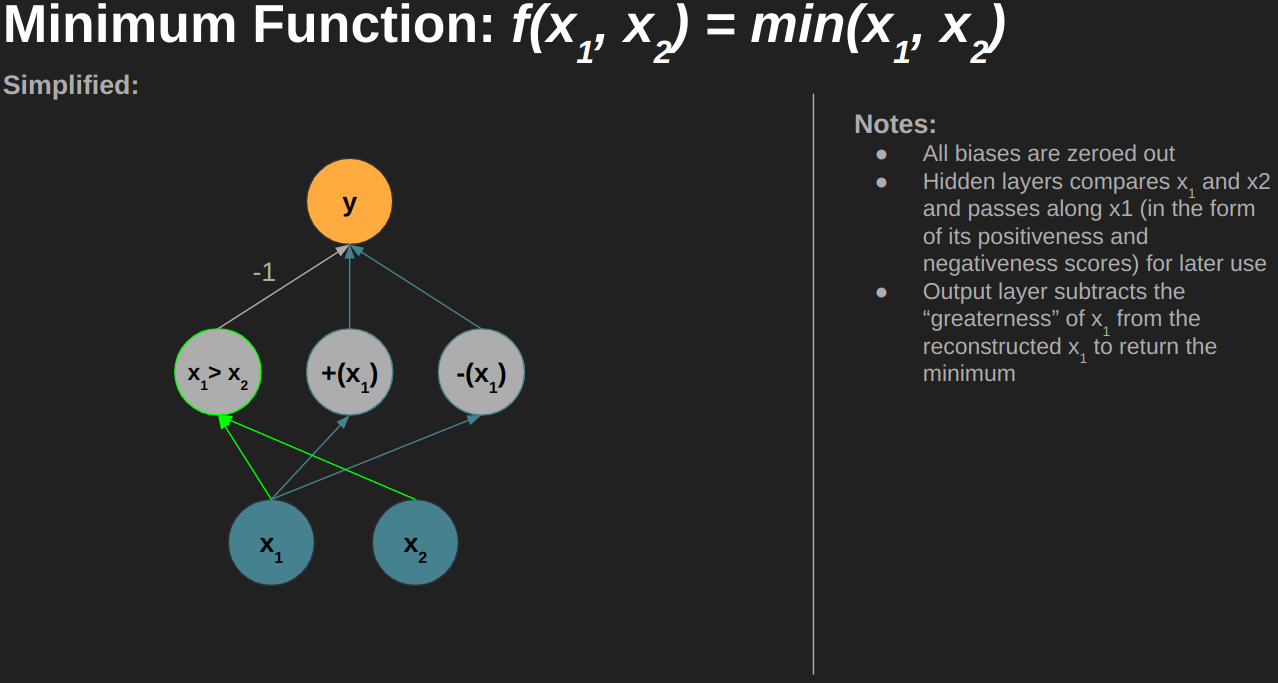# Mathematical Circuits in Neural Networks

1 min read22 Sep 2022 2

# 23

This is one of my final projects for the Columbia EA Summer 2022 Project Based AI Safety Reading Group (special thanks to facilitators Rohan Subramini and Gabe Mukobi). If you're curious you can find my other project here.

## Summary

In this project, I:

1.  Derive by hand the optimal configurations (architecture and weights) of "vanilla" neural networks (multilayer perceptrons; ReLU activations) that implement basic mathematical functions (e.g. absolute value, minimum of two numbers, etc.)
2. Identify "features" and "circuits" of these networks that are reused repeatedly across networks modeling different mathematical functions
3. Verify these theoretical results empirically (in code)

What follows is a brief introduction to this work. For full details, please see:

• The linked video (also embedded at the bottom of this post)
• Or if you prefer to go at your own pace, the slides I walk through in that video

## Motivation

Olah et al. make three claims about the fundamental interpretability of neural networks:They demonstrate these claims in the context of image models:

Features / Circuits:Universality:This work demonstrates the same concepts apply in the space of neural networks modeling basic mathematical functions.

## Results

Specifically, I show that the optimal network for calculating the minimum of two arbitrary numbers is fully constructed from smaller "features" and "circuits" used across even simpler mathematical functions. Along the way, I explore:

• "Positiveness" and "Negativeness" Detectors
• Identity Circuits (i.e. f(x) = x)
• Negative Identity Circuits (i.e. f(x) = -x)
• Subtraction Circuits (i.e. f(x1, x2) = x1 - x2)
• "Greaterness" Detectors
• And More

Minimum Network:I also demonstrate that each of these theoretical results hold in practice. The code for these experiments can be found on the GitHub page for this project.

## Full Details

For full details, please see the PDF presentation in the GitHub repo or watch the full video walkthrough: